Courses

# Number System - Olympiad Level MCQ, Class 9 Mathematics

## 25 Questions MCQ Test Olympiad Preparation for Class 9 | Number System - Olympiad Level MCQ, Class 9 Mathematics

Description
This mock test of Number System - Olympiad Level MCQ, Class 9 Mathematics for Class 9 helps you for every Class 9 entrance exam. This contains 25 Multiple Choice Questions for Class 9 Number System - Olympiad Level MCQ, Class 9 Mathematics (mcq) to study with solutions a complete question bank. The solved questions answers in this Number System - Olympiad Level MCQ, Class 9 Mathematics quiz give you a good mix of easy questions and tough questions. Class 9 students definitely take this Number System - Olympiad Level MCQ, Class 9 Mathematics exercise for a better result in the exam. You can find other Number System - Olympiad Level MCQ, Class 9 Mathematics extra questions, long questions & short questions for Class 9 on EduRev as well by searching above.
QUESTION: 1

### Find the remainder when 73 x 75 x 78 x 57 x 197 x 37 is divided by 34.

Solution:

⇒ (73 x 75 x 78 x 57 x197 x 37) / 34

⇒ (5 x 7 x 10 x 23 x 27 x 3) / 34

[We have taken individual remainder, which means if 73 is divided by 34 individually, it will give remainder 5, 75 divided 34 gives remainder 7 and so on.]

⇒ (5 x 7 x 10 x 23 x 27 x 3)/34

⇒ (35 x 30 x 23 x 27) / 34 [Number Multiplied]
⇒ (35 x 30 x 23 x 27) / 34

⇒ (1 x -4 x -11 x -7) / 34

[We have taken here negative as well as positive remainder at the same time. When 30 divided by 34 it will give either positive remainder 30 or negative remainder -4. We can use any one of negative or positive remainder at any time.]

⇒ (1 x -4 x -11 x  -7) / 34

⇒ (28 x -11) / 34

⇒ (-6 x -11) / 34

⇒  66 / 34

⇒ R = 32.

Required remainder = 32.

QUESTION: 2

Solution:
QUESTION: 3

### The value of x in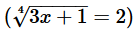is :-

Solution:

(3x+ 1)^1/4 = 2

=> 3x+ 1 = (2²)²

=> 3x+ 1 = 16

=> 3x = 16-1

=> 3x = 15

=> x = 5

QUESTION: 4

Value of (256)0.16 x (256)0.09=?

Solution:

(256)0.16 x (256)0.09 = (256)(0.16 + 0.09)

= (256)0.25

= (256)(25/100)

= (256)(1/4)

= (44)(1/4)

= 44(1/4)

= 41

= 4

QUESTION: 5

Which one of the following is a rational number ?

Solution:

(√2)= 2 which is a rational number

QUESTION: 6

The value of x in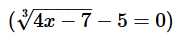is :-

Solution:

(4x -7 )^1/3 -5 =0

(4x -7)^1/3 = 5

4x -7 = 5³

4x -7 =125

4x = 125 +7

4x = 132

x = 132/4

x = 33

QUESTION: 7

Which of the following is not an improper fraction :-

Solution:

In proper fraction, the  numerator is greater than it's denominator whereas in improper fraction denominator is greater than numerator.

QUESTION: 8

Let N = 1421*1423*1425. What is the remainder when N is divided by 12?

Solution:

1421 = 118*12 + 5 = 12k + 5, where k = 118.

1423 = 12k + 7

1425 = 12k + 9

N = (12k + 5)(12 k + 7)(12k + 9)

When N is divided by 12, the remainder is same as the remainder, when 5 * 7 * 9 = 315 is divided by 12.

5 * 7 * 9 = 35 * 9 = 315

315 = 312 + 3 = 12 * 26 + 3

The remainder is 3.

QUESTION: 9

Find the least number which will leaves remainder 5 when divided by 8, 12, 16 and 20.

Solution:

We have to find the Least number, therefore we find out the LCM of 8, 12, 16 and 20.
8 = 2*2*2;
12 = 2*2*3;
16 = 2*2*2*2;
20 = 2*2*5;
LCM = 2*2*2*2*3*5 = 240;
This is the least number which is exactly divisible by 8, 12, 16 and 20.
Thus,
required number which leaves remainder 5 is,
240+5 = 245.

QUESTION: 10

The value of :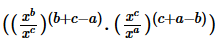.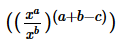Solution:
QUESTION: 11

The binary equivalent of (1011.011)10 is equal to

Solution:

1 * 23 + 0 * 22 + 1 * 21 + 0 * 2^-1 +1 * 2^-2 + 1 * 2^-3 = 11.375
Hence, (1011.011)10 = 11.375

QUESTION: 12

If pqr = 1, then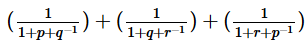is equal to :-

Solution:

LHS = 1/[1 + p + q⁻¹] + 1/[1 + q + r⁻¹] + 1/[1 + r + p⁻¹]

1/[1 + p + q⁻¹] = 1/[1 + p + 1/q] = q/ [q + pq + 1] -------(1)

1/[1 + q + r⁻¹] = 1/[1 + q + 1/r ]
= 1/[1 + q + pqr/r ] {from pqr = 1}
= 1/[1 + q + pq] = 1/[q + pq + 1] --------(2)

1/[1 + r + p⁻¹] = pqr/[pqr + r + pqr/p]
= pqr/[pqr + r + qr] = pq/[pq + 1 + q] ------(3)

Add equations (1), (2) and (3)
q[1 + pq + q] + 1/[q + pq + 1] + PQ/[pq + 1 + q]
= [1 + pq + q]/[1 + pq + q] = 1
Hence , LHS = RHS proved

QUESTION: 13

What is the sum of all two digit numbers that gives a remainder of 3 when they are divided by 7?

Solution:

The two digit number which gives a remainder of 3 when divided by 7 are:

10, 17, 24 94.

Now, these number are in AP series with

1st Term, a = 10;

Number of Terms, n = 13;

Last term, L = 94 and

Common Difference, d = 7.

Sum,

= n*(a+L)/2

= 13*52 = 676.

QUESTION: 14

The exponential form of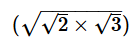is :-

Solution:
QUESTION: 15

If A =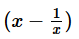, then the value of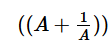is :-

Solution:
QUESTION: 16

If x =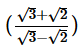and y = 1, the value of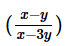is :-

Solution:
QUESTION: 17

If a =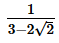, b =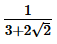then value of a3 + b3 is :-

Solution:
QUESTION: 18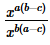÷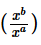=

Solution:
QUESTION: 19

If 2x = 3y = 6-z, then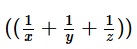is equal to :-

Solution: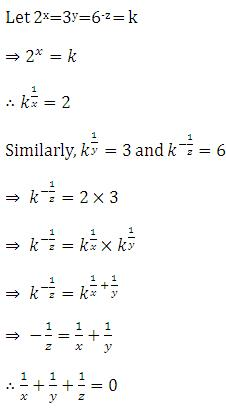QUESTION: 20

If ax = b, by = c and cz = a, then the value of xyz is :-

Solution:

From the following, we need to find the values of x,y, and z:

a x =b , b y =c , c z =a

From ax =  b, we get x  =b/a -------------------------- (i)
From by = c, we get y  =c/b ---------------------------(ii)
From cz = a, we get z = c/a ---------------------------(iii)

Multiplying the left hand sides and right hand sides of (i), (ii) and (iii) we get
xyz=(b/a)*(c/b)*(c/a)

When we simplify, we find that xyz=1. Hence proved.

QUESTION: 21

Solution set of the equation |x – 2| = 5 is :-

Solution:
QUESTION: 22

Three numbers are in ratio 1:2:3 and HCF is 12. The numbers are:

Solution:

Let the required numbers be x, 2x and 3x. Then, their H.C.F. = x. So, x = 12  ∴  The numbers are 12, 24 and 36

QUESTION: 23

The minimum value of the expression |17x – 8 | – 9 is :-

Solution:

Let's say the following polynomial be f(x) .

f(x) = | 17x - 8 | - 9 .

We know that Modulus value can be 0 at least and at most positive infinite.

So, | 17x - 8 | = 0

17x - 8 = 0
x = 8/17 .

Now, Finding

f min ( x) = | 17(8/17)-8 | - 9
= 0 - 9
= -9.

Therefore, The minimum value of | 17x - 8 | - 9 is -9.

QUESTION: 24

If 2a – 9 = b + a, then the value of (|a – b| + |b – a|) is :-

Solution:
QUESTION: 25

If the decimal number is a fraction then its binary equivalent is obtained by ________ the number continuously by 2.

Solution:

On multiplying the decimal number continuously by 2, the binary equivalent is obtained by the collection of the integer part. However, if it’s an integer, then it’s binary equivalent is determined by dividing the number by 2 and collecting the remainders.